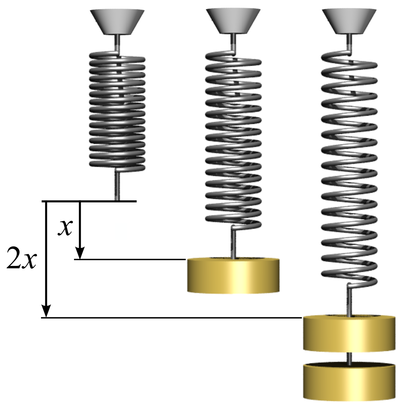# Work Done

## Key Stage 3

### Meaning

Work Done is the amount of energy transferred by the action of a force.

Work Done is measured in Joules.
Work Done can only be calculated for objects moving in the direction of the force. If the direction of the force and the motion of an object are perpendicular then no work is being done on the object by that force.

### Equation

Work Done = (Force) x (Distance moved in the direction of the force)

$$W = F \times d$$

$$W = Fd$$

Work done is written with a capital W. Force is written with a capital F. Distance is written with a lower case d.

### Example Calculations

 A person lifts a 40N box by a height of 1.2m. Calculate the work done by the person against gravity. The brakes on a car apply a force of 10,000N. The car travels a distance of 15m braking. Calculate the work done by the brakes. A person carries a 20N box along a horizontal path of 20m. Force = 40N Distance moved in the direction of the force = 1.2m $$W = Fd$$ $$W = 40 \times 1.2$$ $$W = 48J$$ Force = 10,000N Distance moved in the direction of the force = 15m $$W = Fd$$ $$W = 10,000 \times 15$$ $$W = 15,000J$$ Force = 40N Distance moved in the direction of the force = 0m $$W = Fd$$ $$W = 20 \times 0$$ $$W = 0J$$ No work has been done because the movement is not in the direction of the force. The weight acts downwards but the movement was horizontal.

### Work Done by Deformation

When an object is deformed, such as a spring being stretched, work is done to transfer energy into its Elastic Potential Energy Store.

#### Work Done on a SpringWork is done to stretch this spring.

The work done on a spring can be found with the following equation

$$W = \frac{1}{2} kx^2$$

Where:

W = Work Done

x = Extension of the object.

## Key Stage 4

### Meaning

Work Done is the amount of energy transferred by the action of a force.

Work Done is measured in Joules.

#### Work Done by a Force

Work Done by a force can only be calculated for objects moving in the direction of the force. If the direction of the force and the motion of an object are perpendicular then no work is being done on the object by that force.

#### Work Done by Electricity

Work can also be done by an electrical current as the electrostatic field inside a wire provides a force to move the electrons (or ions).

### Equation for Work Done by a Force

Work Done = (Force) x (Distance moved in the direction of the force)

$$W = Fd$$

Where:

W = Work done

F = The Force applied to the object.

d = The distance moved by the object in the direction of the force.

### Equation for Work Done by Electricity

Work Done = (Current) x (Potential Difference) x (time)

$$W = IVt$$

Where:

W = Work done

I = The current through the component

V = The potential difference across the component.

t = The length of time that the current is flowing.

NB: You do not need to remember this equation. However you do need to remember the two equations which are combined to make this equation.

1. $$P = IV$$

2. $$W = Pt$$

Where:

P = Power

Equation 1 is substituted into equation 2 to give $$W = IVt$$.

### Equation for Work Done by Deformation

$$W = \frac{1}{2} kx^2$$

Where:

W = Work Done

x = Extension of the object.